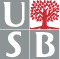MAT 536 Algebra III - Algebraic Geometry: An Introduction

Instructor    Sorin Popescu   (office: Math 4-119, tel. 632-8358, e-mail sorin@math.sunysb.edu)

 Prerequisites

A solid foundation in abstract algebra, linear algebra and field theory (such as the first year graduate courses in algebra MAT 534 and MAT 535), and perhaps some knowledge of elementary commutative algebra/algebraic geometry, topology, and complex analysis. MAT 536 is designed as an introductory course and I will try to keep the amount of required previous knowledge to a minimum.

 Textbook(s)

 Algebraic Geometry: A first course, by Joe Harris, GTM 133, Springer Verlag 1995. This is an example-oriented book which gives a gentle introduction to classical algebraic geometry. Writen in an informal style it is intended for a first course in algebraic geometry and is recommended to graduate students and possibly some advanced undergraduates who have had extensive experience in abstract algebra. The first part of the book is concerned with introducing basic varieties and constructions; it describes, for example, affine and projective varieties, regular and rational maps, and particular classes of varieties such as determinantal varieties and algebraic groups. The second part discusses attributes of varieties, including dimension, smoothness, tangent spaces and cones, degree, and parameter and moduli spaces. Other recommended texts: Basic Algebraic Geometry 1, and Basic Algebraic Geometry 2, I.R. Shafarevich, Springer Verlag 1995 Principles of Algebraic Geometry, Phillip Griffiths and Joseph Harris, John Wiley & Sons, 1994 Algebraic Geometry, Robin Hartshorne, GTM 52, Springer Verlag 1997 The Geometry of Schemes, David Eisenbud and Joe Harris, GTM 197, Springer Verlag 1999Course description

We will try to cover the topics in both Part I (Examples of Varieties and Maps), and Part II (Attributes of Varieties) of the textbook. The spirit of the book is to study a substantial amount of examples without developing extensive amounts of theory, while minimizing the technical algebraic prerequisities. This approach may seem somewhat unusual, but it works well for learning algebraic geometry - especially if one wants to have a good intuitive grasp of what the theorems really mean.

We may skip around a bit especially for the first few weeks:

• Algebraic Varieties in Kn.  Projective space KPn.(pp. 3-5)
• Projective hyperplanes, linear subspaces, and varieties. (pp. 3-8). A statement of Bezout's Theorem. (p. 173)
• Counting tangent lines to a fixed conic from a fixed point.
• Conics simultaneously containing 5 points (p. 12) or tangent to 5 lines.
• Gauss map of a planar curve. (p. 188)
• Ideals of varieties (p.48), Hilbert Basis theorem, K[x1, ... , xn]  is Noetherian.
• The Zariski  topology (pp. 17-18). Finite sets as algebraic varieties (pp. 6-7).
• The Twisted Cubic, Rational Normal Curves(pp. 9-10)
• Complete and set-theoretic intersections (pp. 136-137)
• Hilbert's Nullstellensatz and consequences (pp. 48-62)
• Regular Maps (pp. 21-25)
• Cones, projections, and products (pp. 31-40)
• Definitions of dimension(pp. 133-135)
• Regularity of Zariski open dense subset of a complex hypersurface
• Rational functions and maps (pp. 72-81)
• Grassmannians and related varieties (pp. 63-68)
• Incidence and secant varieties (pp. 142-143)
• Algebraic Groups (pp. 114-123)
• Smoothness and Zariski tangent space (pp. 174-177)
• Stratifications of complex varieties
• Projective tangent spaces (pp. 181-183)
• Degree and Bezout's theorem (pp. 224-229), (pp. 235-237)
• Parameter spaces and Moduli spaces (pp. 266-273)

 Homework

I will assign problems in each lecture, ranging in difficulty from routine to more challenging. Course grades will be based on these problems (and any other participation); solving at least half of them will be considered a perfect score.

 Software

Concrete problems such as the study of certain explicitly given (i.e. by equations) algebraic varieties can be successfully attacked with the help of specialized computer algebra systems:

Sorin Popescu

2001-08-17